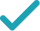Type a math problemSolve for bSteps for Solving Linear Equation
Swap sides so that all variable terms are on the left hand side.
Subtract from both sides.
Subtract from to get .
The equation is in standard form.
Divide both sides by .
Dividing by undoes the multiplication by .
Divide by .
Solve for xSteps for Solving Linear Equation
Subtract from both sides.
Subtract from both sides.
Subtract from to get .
Combine all terms containing .
The equation is in standard form.
Divide both sides by .
Dividing by undoes the multiplication by .
Divide by .
GraphGraph Both Sides in 2D
Graph in 2DGiving is as easy as 1, 2, 3
Get 1,000 points to donate to a school of your choice when you join Give With Bing
bx+\frac{1}{3}=\frac{2}{3}-5x
Swap sides so that all variable terms are on the left hand side.
bx=\frac{2}{3}-5x-\frac{1}{3}
Subtract \frac{1}{3}\approx 0.333333333 from both sides.
bx=\frac{1}{3}-5x
Subtract \frac{1}{3}\approx 0.333333333 from \frac{2}{3}\approx 0.666666667 to get \frac{1}{3}\approx 0.333333333.
xb=\frac{1}{3}-5x
The equation is in standard form.
\frac{xb}{x}=\frac{\frac{1}{3}-5x}{x}
Divide both sides by x.
b=\frac{\frac{1}{3}-5x}{x}
Dividing by x undoes the multiplication by x.
b=-5+\frac{1}{3x}
Divide \frac{1}{3}-5x by x.
\frac{2}{3}-5x-bx=\frac{1}{3}
Subtract bx from both sides.
-5x-bx=\frac{1}{3}-\frac{2}{3}
Subtract \frac{2}{3}\approx 0.666666667 from both sides.
-5x-bx=-\frac{1}{3}
Subtract \frac{2}{3}\approx 0.666666667 from \frac{1}{3}\approx 0.333333333 to get -\frac{1}{3}\approx -0.333333333.
\left(-5-b\right)x=-\frac{1}{3}
Combine all terms containing x.
\left(-b-5\right)x=-\frac{1}{3}
The equation is in standard form.
\frac{\left(-b-5\right)x}{-b-5}=\frac{-\frac{1}{3}}{-b-5}
Divide both sides by -5-b.
x=\frac{-\frac{1}{3}}{-b-5}
Dividing by -5-b undoes the multiplication by -5-b.
x=\frac{1}{3\left(b+5\right)}
Divide -\frac{1}{3}\approx -0.333333333 by -5-b.